# sklearn.gaussian_process.GaussianProcessClassifier¶

class sklearn.gaussian_process.GaussianProcessClassifier(kernel=None, optimizer=’fmin_l_bfgs_b’, n_restarts_optimizer=0, max_iter_predict=100, warm_start=False, copy_X_train=True, random_state=None, multi_class=’one_vs_rest’, n_jobs=None)[source]

Gaussian process classification (GPC) based on Laplace approximation.

The implementation is based on Algorithm 3.1, 3.2, and 5.1 of Gaussian Processes for Machine Learning (GPML) by Rasmussen and Williams.

Internally, the Laplace approximation is used for approximating the non-Gaussian posterior by a Gaussian.

Currently, the implementation is restricted to using the logistic link function. For multi-class classification, several binary one-versus rest classifiers are fitted. Note that this class thus does not implement a true multi-class Laplace approximation.

Parameters: kernel : kernel object The kernel specifying the covariance function of the GP. If None is passed, the kernel “1.0 * RBF(1.0)” is used as default. Note that the kernel’s hyperparameters are optimized during fitting. optimizer : string or callable, optional (default: “fmin_l_bfgs_b”) Can either be one of the internally supported optimizers for optimizing the kernel’s parameters, specified by a string, or an externally defined optimizer passed as a callable. If a callable is passed, it must have the signature: def optimizer(obj_func, initial_theta, bounds): # * 'obj_func' is the objective function to be maximized, which # takes the hyperparameters theta as parameter and an # optional flag eval_gradient, which determines if the # gradient is returned additionally to the function value # * 'initial_theta': the initial value for theta, which can be # used by local optimizers # * 'bounds': the bounds on the values of theta .... # Returned are the best found hyperparameters theta and # the corresponding value of the target function. return theta_opt, func_min  Per default, the ‘fmin_l_bfgs_b’ algorithm from scipy.optimize is used. If None is passed, the kernel’s parameters are kept fixed. Available internal optimizers are: 'fmin_l_bfgs_b'  n_restarts_optimizer : int, optional (default: 0) The number of restarts of the optimizer for finding the kernel’s parameters which maximize the log-marginal likelihood. The first run of the optimizer is performed from the kernel’s initial parameters, the remaining ones (if any) from thetas sampled log-uniform randomly from the space of allowed theta-values. If greater than 0, all bounds must be finite. Note that n_restarts_optimizer=0 implies that one run is performed. max_iter_predict : int, optional (default: 100) The maximum number of iterations in Newton’s method for approximating the posterior during predict. Smaller values will reduce computation time at the cost of worse results. warm_start : bool, optional (default: False) If warm-starts are enabled, the solution of the last Newton iteration on the Laplace approximation of the posterior mode is used as initialization for the next call of _posterior_mode(). This can speed up convergence when _posterior_mode is called several times on similar problems as in hyperparameter optimization. See the Glossary. copy_X_train : bool, optional (default: True) If True, a persistent copy of the training data is stored in the object. Otherwise, just a reference to the training data is stored, which might cause predictions to change if the data is modified externally. random_state : int, RandomState instance or None, optional (default: None) The generator used to initialize the centers. If int, random_state is the seed used by the random number generator; If RandomState instance, random_state is the random number generator; If None, the random number generator is the RandomState instance used by np.random. multi_class : string, default Specifies how multi-class classification problems are handled. Supported are “one_vs_rest” and “one_vs_one”. In “one_vs_rest”, one binary Gaussian process classifier is fitted for each class, which is trained to separate this class from the rest. In “one_vs_one”, one binary Gaussian process classifier is fitted for each pair of classes, which is trained to separate these two classes. The predictions of these binary predictors are combined into multi-class predictions. Note that “one_vs_one” does not support predicting probability estimates. n_jobs : int or None, optional (default=None) The number of jobs to use for the computation. None means 1 unless in a joblib.parallel_backend context. -1 means using all processors. See Glossary for more details. kernel_ : kernel object The kernel used for prediction. In case of binary classification, the structure of the kernel is the same as the one passed as parameter but with optimized hyperparameters. In case of multi-class classification, a CompoundKernel is returned which consists of the different kernels used in the one-versus-rest classifiers. log_marginal_likelihood_value_ : float The log-marginal-likelihood of self.kernel_.theta classes_ : array-like, shape = (n_classes,) Unique class labels. n_classes_ : int The number of classes in the training data

Examples

>>> from sklearn.datasets import load_iris
>>> from sklearn.gaussian_process import GaussianProcessClassifier
>>> from sklearn.gaussian_process.kernels import RBF
>>> kernel = 1.0 * RBF(1.0)
>>> gpc = GaussianProcessClassifier(kernel=kernel,
...         random_state=0).fit(X, y)
>>> gpc.score(X, y)
0.9866...
>>> gpc.predict_proba(X[:2,:])
array([[0.83548752, 0.03228706, 0.13222543],
[0.79064206, 0.06525643, 0.14410151]])


New in version 0.18.

Methods

 fit(self, X, y) Fit Gaussian process classification model get_params(self[, deep]) Get parameters for this estimator. log_marginal_likelihood(self[, theta, …]) Returns log-marginal likelihood of theta for training data. predict(self, X) Perform classification on an array of test vectors X. predict_proba(self, X) Return probability estimates for the test vector X. score(self, X, y[, sample_weight]) Returns the mean accuracy on the given test data and labels. set_params(self, \*\*params) Set the parameters of this estimator.
__init__(self, kernel=None, optimizer=’fmin_l_bfgs_b’, n_restarts_optimizer=0, max_iter_predict=100, warm_start=False, copy_X_train=True, random_state=None, multi_class=’one_vs_rest’, n_jobs=None)[source]
fit(self, X, y)[source]

Fit Gaussian process classification model

Parameters: X : array-like, shape = (n_samples, n_features) Training data y : array-like, shape = (n_samples,) Target values, must be binary self : returns an instance of self.
get_params(self, deep=True)[source]

Get parameters for this estimator.

Parameters: deep : boolean, optional If True, will return the parameters for this estimator and contained subobjects that are estimators. params : mapping of string to any Parameter names mapped to their values.
log_marginal_likelihood(self, theta=None, eval_gradient=False)[source]

Returns log-marginal likelihood of theta for training data.

In the case of multi-class classification, the mean log-marginal likelihood of the one-versus-rest classifiers are returned.

Parameters: theta : array-like, shape = (n_kernel_params,) or none Kernel hyperparameters for which the log-marginal likelihood is evaluated. In the case of multi-class classification, theta may be the hyperparameters of the compound kernel or of an individual kernel. In the latter case, all individual kernel get assigned the same theta values. If None, the precomputed log_marginal_likelihood of self.kernel_.theta is returned. eval_gradient : bool, default: False If True, the gradient of the log-marginal likelihood with respect to the kernel hyperparameters at position theta is returned additionally. Note that gradient computation is not supported for non-binary classification. If True, theta must not be None. log_likelihood : float Log-marginal likelihood of theta for training data. log_likelihood_gradient : array, shape = (n_kernel_params,), optional Gradient of the log-marginal likelihood with respect to the kernel hyperparameters at position theta. Only returned when eval_gradient is True.
predict(self, X)[source]

Perform classification on an array of test vectors X.

Parameters: X : array-like, shape = (n_samples, n_features) C : array, shape = (n_samples,) Predicted target values for X, values are from classes_
predict_proba(self, X)[source]

Return probability estimates for the test vector X.

Parameters: X : array-like, shape = (n_samples, n_features) C : array-like, shape = (n_samples, n_classes) Returns the probability of the samples for each class in the model. The columns correspond to the classes in sorted order, as they appear in the attribute classes_.
score(self, X, y, sample_weight=None)[source]

Returns the mean accuracy on the given test data and labels.

In multi-label classification, this is the subset accuracy which is a harsh metric since you require for each sample that each label set be correctly predicted.

Parameters: X : array-like, shape = (n_samples, n_features) Test samples. y : array-like, shape = (n_samples) or (n_samples, n_outputs) True labels for X. sample_weight : array-like, shape = [n_samples], optional Sample weights. score : float Mean accuracy of self.predict(X) wrt. y.
set_params(self, **params)[source]

Set the parameters of this estimator.

The method works on simple estimators as well as on nested objects (such as pipelines). The latter have parameters of the form <component>__<parameter> so that it’s possible to update each component of a nested object.

Returns: self

## Examples using sklearn.gaussian_process.GaussianProcessClassifier¶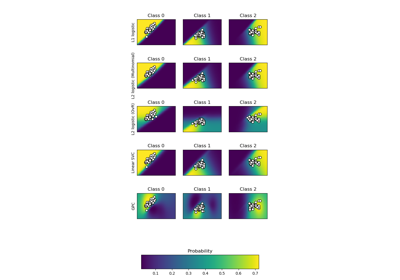Plot classification probability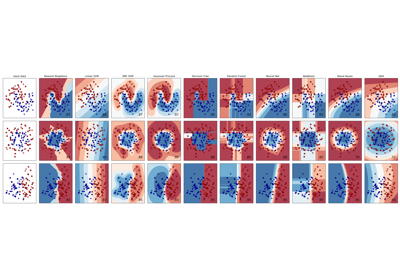Classifier comparison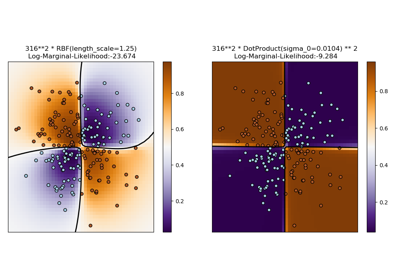Illustration of Gaussian process classification (GPC) on the XOR dataset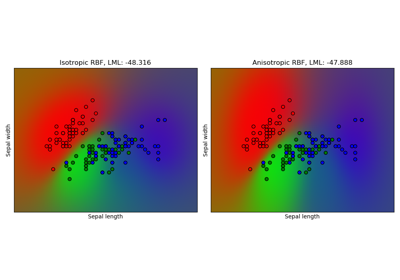Gaussian process classification (GPC) on iris dataset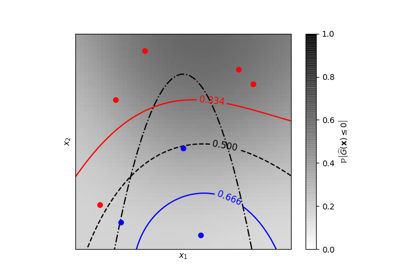Iso-probability lines for Gaussian Processes classification (GPC)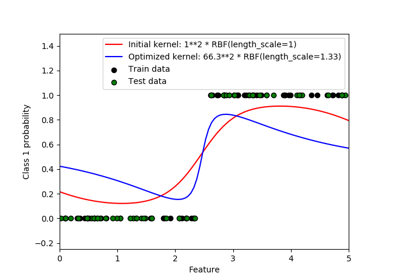Probabilistic predictions with Gaussian process classification (GPC)